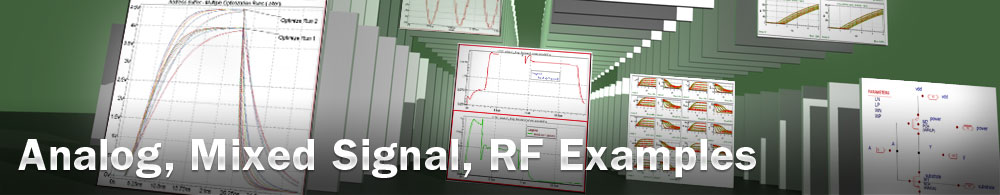RF Frequency Divider Schematic Simulation

## 003_freq_divider : RF Frequency Divider Schematic Simulation

Minimum Required Versions: Gateway 2.12.8.R, SmartSpiceRF 1.6.3.R

The schematic for Example 3 ( ) is a frequency divider design. Since the division factor is 4, the fundamental frequency is set to the output frequency (FUND1=250MegHz) equal to the input frequency (1 GHz) divided by 4. RF input source at 4*FUND1frequency is specified as fourth harmonic stimuli: Vck ck GND DC 0.9 MTS1(0.9 0.0 4) .

In the control file ( freq_divider.ctr), a large number of harmonics (nharm1=40) and an oversampling factor of 2 (oversample=2) are specified to better represent the square-like waveforms produced by this nonlinear circuit.

A Transient Initial Guess (TIG) is set by UseTIG=3. Transient simulation time is defined by TIGtstop=5n. The accuracy option reltol=1e-6 is set to obtain an accurate initial guess.

The quality of the initial guess can be determined by checking the first Newton KCL residual (displayed with annotate=4). The lower the residual numerical value, the better is the initial guess.

The .IPLOT harm_sp vdb0 vdb1 statement is used to interactively plot the spectra for b0 and b1 as the simulation runs. The resulting plot is shown in SmartView ( ).

Expanding the transient analysis in the data browser, the waveforms are plotted for nodes b0 and b1 ( ).

Finally, the RF input stimulus, the RF voltage waveform at node b0 (division by 2), and the RF voltage waveform at node b1 (division by 4) are seen on the RF plot ( ).